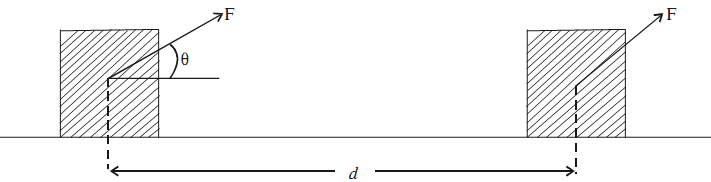# Work

Suppose that a constant force F acting on an object results in displacement d i.e. moves it by a distance d along a straight line on a horizontal surface. The work done by a force is the product of the magnitude of force component in the direction of displacement and the displacement of this object.If force F is acting at angle θ with respect to the displacement d of the object, its component along d will be F cosθ. Then work done by force F is given by

W = F cosθ.d

In vector form, the work done is given by

W = F. d

If d = 0, W = 0. That is, no work is done by a force, whatever its magnitude, if there is no displacement of the object.

### Unit of Work

If the applied force is in newton and displacement is in metre, then the unit of work is joule. One joule is defined, as the work done by a force of one newton when it produces a displacement of one metre. Joule is the SI unit of work.